## Two Particles in Three Dimensions

The generalization of the Hamiltonian to three dimensions is simple.We define the vector difference between the coordinates of the particles.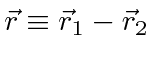We also define the vector position of the center of mass.We will use the chain rule to transform our Hamiltonian. As a simple example, if we were working in one dimension we might use the chain rule like this.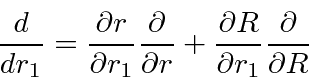In three dimensions we would have.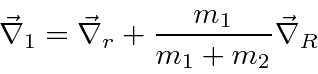Putting this into the Hamiltonian we get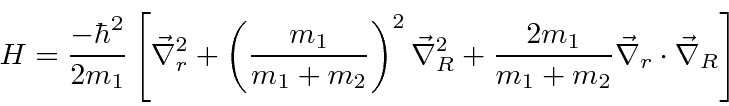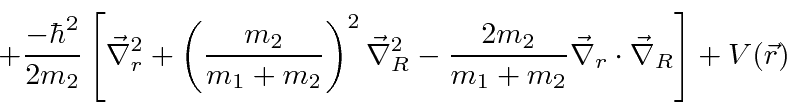Defining the reduced mass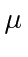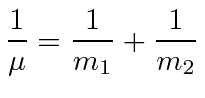and the total masswe get.The Hamiltonian actually separates into two problems: the motion of the center of mass as a free particleand the interaction between the two particles.This is exactly the same separation that we would make in classical physics.

Jim Branson 2013-04-22